# RS Aggarwal Class 7 Solutions Chapter 20 Mensuration Ex 20G

## RS Aggarwal Class 7 Solutions Chapter 20 Mensuration Ex 20G

These Solutions are part of RS Aggarwal Solutions Class 7. Here we have given RS Aggarwal Solutions Class 7 Chapter 20 Mensuration Ex 20G.

Other Exercises

Objective Questions
Mark (✓) against the correct answer in each of the following :
Question 1.
Solution:
(c)
Length of rectangle AB = 16 cm
and diagonal BD = 20 cm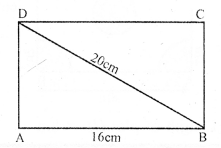But, in right ∆ABD
BD² = AB² + AD²
⇒ (20)² = (16)² + AD²
⇒ 400 = 256 + AD²
⇒ AD² = 400 – 256 = 144 = (12)²
⇒ AD = 12 cm
Area = l x b = 16 x 12 = 192 cm²

Question 2.
Solution:
(b)
Diagonal of square = 12 cm
Let side = 9
diagonal = √2 a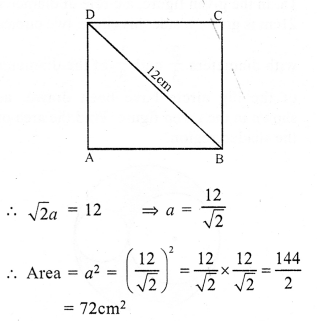Question 3.
Solution:
(b)
Area = 200 cm²
side = √200 =√2 x 10
and diagonal = √2 a = √2 x √2 x 10 = 20

Question 4.
Solution:
(a)
Area of square = 0.5 hectare = 0.5 x 10000 = 5000 m²
= √10000 = 100 m

Question 5.
Solution:
(c)
Perimeter of rectangle = 240m
l + b = $$\frac { 240 }{ 2 }$$ = 120 m
Let breadth = x, then length = 3x .
3x + x = 120
⇒ 4x = 120
⇒ x = 30
Length = 3x = 3 x 30 = 90 m

Question 6.
Solution:
Let original side of square = x
area = x²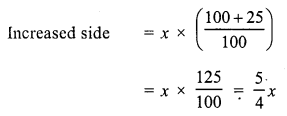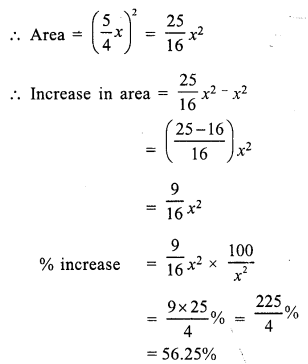Question 7.
Solution:
(b)
Let side of square = a
Then its diagonal = √2 a
Now, area of square = a²
and area of square on diagonal = (√2 a)² = 2a²
Ratio = a² : 2a² = 1 : 2

Question 8.
Solution:
(c)
If perimeters of a square and a rectangle are equal Then the area of the square will be greater than that of a rectangle
A > B

Question 9.
Solution:
(b)
Perimeter of rectangle = 480m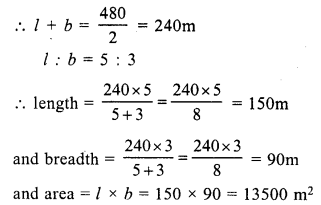Question 10.
Solution:
(a)
Total cost of carpet = Rs. 6000
Rate per metre = Rs. 50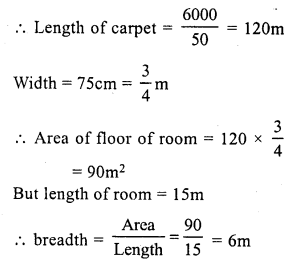Question 11.
Solution:
(a)
Sides are 13 cm, 14 cm, 15 cm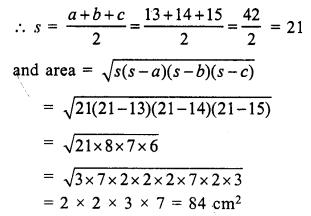Question 12.
Solution:
(b)
Base of triangle = 12 m
and height = 8m
Area= $$\frac { 1 }{ 2 }$$ x Base x height
= $$\frac { 1 }{ 2 }$$ x 12 x 8 = 48 m²

Question 13.
Solution:
(b)
Let side = a
then area = $$\frac { \surd 3 }{ 4 }$$ a²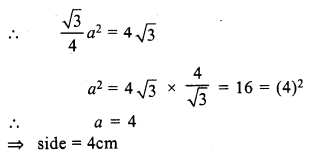Question 14.
Solution:
(c)
Side of an equilateral triangle = 8cm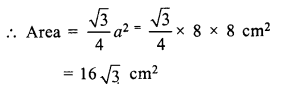Question 15.
Solution:
(b)
Let a be the side of an equilateral triangle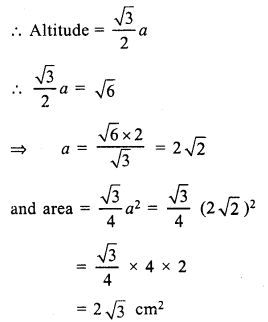Question 16.
Solution:
(b)
One side (Base) of parallelogram = 16 cm
and altitude = 4.5 cm
Area = base x altitude = 16 x 4.5 = 72 cm²

Question 17.
Solution:
(b)
Length of diagonals of a rhombus are 24 cm and 18 cm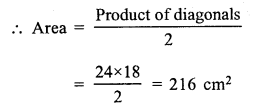Question 18.
Solution:
(c)
Let r be the radius of the circle Then
c = 2πr
2πr – r = 37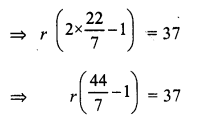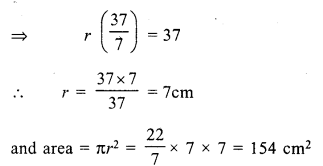Question 19.
Solution:
(c) Perimeter of room = 18 m
and height = 3 m
Area of 4 walls = Perimeter x height = 18 x 3 = 54 m²

Question 20.
Solution:
(a) Area of floor = l x b = 14 x 9 = 126 m²
Area of carpet = 126 m²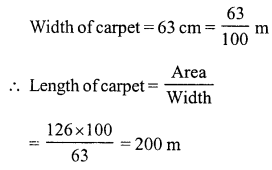Question 21.
Solution:
(c)
Perimeter = 46 cm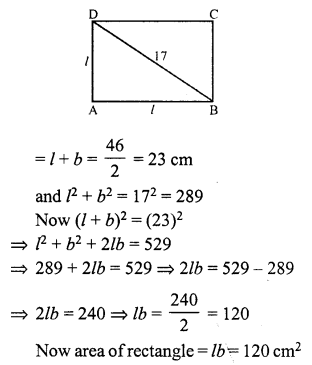Question 22.
Solution:
(b)
Ratio in area of two squares = 9 : 1
Let area of bigger square = 9x²
and of smaller square = x²
Side of bigger square = √9x² = 3x
and perimeter = 4 x side = 4 x 3x = 12x
Side of smaller square = √x² = x
Perimeter = 4x
Now ratio in their perimeter = 12x : 4x = 3 : 1

Question 23.
Solution:
(d)
Let the diagonals of two square be 2d and d
Area of bigger square 2 (2d)² = 8d²
and of smaller = 2 (d)² = 2d²
Ratio in their area = $$\frac { 8{ d }^{ 2 } }{ 2{ d }^{ 2 } } =\frac { 4 }{ 1 }$$
= 4 : 1

Question 24.
Solution:
(c)
Side of square = 84 m
Area of square = (84)² = 7056 m²
Area of rectangle = 7056 m²
Length of rectangle = 144 m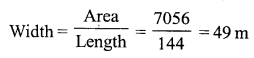Question 25.
Solution:
(d)
Side of a square = a
Area = a²
Side of equilateral triangle = a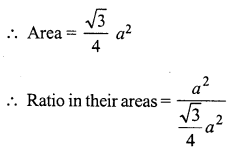Question 26.
Solution:
(a)
Let a be the side of a square
Area = a²
Then area of circle = a²
Let r be the radius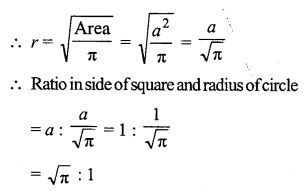Question 27.
Solution:
(b)
Let each side of an equilateral triangle = a
Then area = $$\frac {\surd 3 }{ 4 }$$ a²
Now radius of the circle = a
Then area = πr² = πa²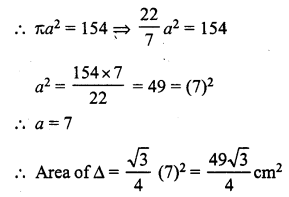Question 28.
Solution:
(c)
Area of rhombus = 36 cm²
Length of one diagonal = 6 cm
Length of second diagonal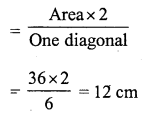Question 29.
Solution:
(d)
Area of a rhombus =144 cm²
Let one diagonal (d1) = a
then Second diagonal (d2) = 2a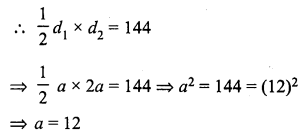Larger diagonal = 2a = 2 x 12 = 24 cm

Question 30.
Solution:
(c)
Area of a circle = 24.64 m²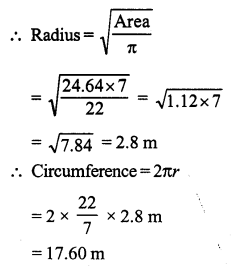Question 31.
Solution:
(c)
Let original radius = r
Then its area = πr²
Radius of increased circle = r + 1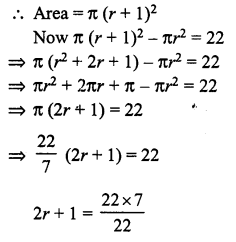2r = 7 – 1 = 6
⇒ r = $$\frac { 6 }{ 2 }$$ = 3
Radius of original circle = 3 cm

Question 32.
Solution:
(c)
Radius of a circular wheel (r) = 1.75 m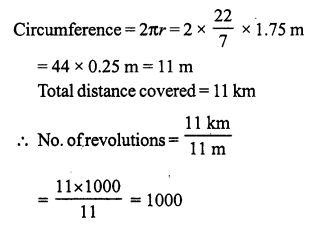Hope given RS Aggarwal Solutions Class 7 Chapter 20 Mensuration Ex 20G are helpful to complete your math homework.

If you have any doubts, please comment below. Learn Insta try to provide online math tutoring for you.